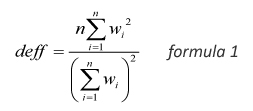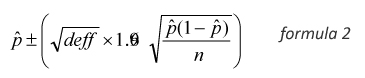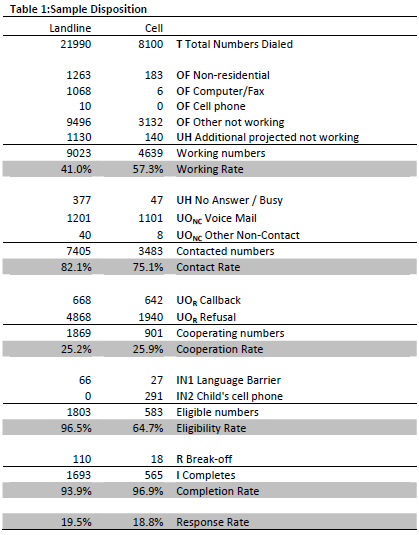### Summary

This report is based on the findings of a daily tracking survey on Americans’ use of the Internet. The results in this report are based on data from telephone interviews conducted by Princeton Survey Research Associates International between November 30 and December 27, 2009, among a sample of 2,258 adults, age 18 and older.  Interviews were conducted in both English (n=2,197) and Spanish (n=61).  Statistical results are weighted to correct known demographic discrepancies.

The margin of sampling error for the complete set of weighted data (n=2,258) is ±2 percentage points. Margins of error for subgroups may be larger than the margin of error for the total sample. For example, the findings for internet users are based on a subsample of 1,676 adults and have a margin of error of ±3 percentage points; the findings for online government users are based on a subsample of 1,375 adults and have a margin of error of ±3 percentage points. Sampling errors and statistical tests of significance in this study take into account the effect of weighting (described below).

A combination of landline and cellular random digit dial (RDD) samples was used to represent all adults in the continental United States who have access to either a landline or cellular telephone. Both samples were provided by Survey Sampling International, LLC (SSI) according to PSRAI specifications.  Numbers for the landline sample were selected with probabilities in proportion to their share of listed telephone households from active blocks (area code + exchange + two-digit block number) that contained three or more residential directory listings. The cellular sample was not list-assisted, but was drawn through a systematic sampling from dedicated wireless 100-blocks and shared service 100-blocks with no directory-listed landline numbers.

Non-response in telephone interviews produces some known biases in survey-derived estimates because participation tends to vary for different subgroups of the population, and these subgroups are likely to vary also on questions of substantive interest. In order to compensate for these known biases, the sample data are weighted in analysis. The demographic weighting parameters are derived from a special analysis of the most recently available Census Bureau’s March 2009 Annual Social and Economic Supplement. This analysis produces population parameters for the demographic characteristics of adults age 18 or older. These parameters are then compared with the sample characteristics to construct sample weights. The weights are derived using an iterative technique that simultaneously balances the distribution of all weighting parameters.

### Quantitative Survey Design and Data Collection Procedures

Effects of Sample Design on Statistical Inference

Post-data collection statistical adjustments require analysis procedures that reflect departures from simple random sampling. PSRAI calculates the effects of these design features so that an appropriate adjustment can be incorporated into tests of statistical significance when using these data. The so-called “design effect” or deff represents the loss in statistical efficiency that results from systematic non-response. The total sample design effect for this survey is 1.18.

PSRAI calculates the composite design effect for a sample of size n, with each case having a weight, wi as:In a wide range of situations, the adjusted standard error of a statistic should be calculated by multiplying the usual formula by the square root of the design effect (√deff ). Thus, the formula for computing the 95% confidence interval around a percentage is:where p^ is the sample estimate and n is the unweighted number of sample cases in the group being considered.

The survey’s margin of error is the largest 95% confidence interval for any estimated proportion based on the total sample— the one around 50%. For example, the margin of error for the entire sample is ±3.8%. This means that in 95 out every 100 samples drawn using the same methodology, estimated proportions based on the entire sample will be no more than 3.8 percentage points away from their true values in the population. This margin of error takes into account the potential design effect of weighting. A multiplier of 1.2 (the square root of the typical design effect of the weight variable) is included in Pew Internet’s margin of error formula:

1.2*1.96*(SQRT(.5 * .5) / N)*100

It is important to remember that sampling fluctuations are only one possible source of error in a survey estimate. Other sources, such as respondent selection bias, questionnaire wording and reporting inaccuracy, may contribute additional error of greater or lesser magnitude.

Response Rate

Table 2 reports the disposition of all sampled callback telephone numbers ever dialed. The response rate estimates the fraction of all eligible respondents in the sample that were ultimately interviewed. At PSRAI it is calculated by taking the product of three component rates:5

• Contact rate – the proportion of working numbers where a request for interview was made
• Cooperation rate – the proportion of contacted numbers where a consent for interview was at least initially obtained, versus those refused
• Completion rate – the proportion of initially cooperating and eligible interviews that agreed to the child interview and were completed

Thus the response rate for the landline sample was 19.5 percent. The response rate for the cellular sample was 18.8 percent.